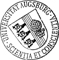## On the complete classification of unitary N = 2 minimal superconformal field theories

• Aiming at a complete classification of unitary N = 2 minimal models (where the assumption of space-time supersymmetry has been dropped), it is shown that each candidate for a modular invariant partition function of such a theory is indeed the partition function of a minimal model. A family of models constructed via orbifoldings of either the diagonal model or of the space-time supersymmetric exceptional models demonstrates that there exists a unitary N = 2 minimal model for every one of the allowed partition functions in the list obtained from Gannon's work [Gannon1996]. Kreuzer and Schellekens' conjecture that all simple current invariants can be obtained as orbifolds of the diagonal model, even when the extra assumption of higher-genus modular invariance is dropped, is confirmed in the case of the unitary N = 2 minimal models by simple counting arguments. We find a nice characterisation of the projection from the Hilbert space of a minimal model with k odd to its modular invariantAiming at a complete classification of unitary N = 2 minimal models (where the assumption of space-time supersymmetry has been dropped), it is shown that each candidate for a modular invariant partition function of such a theory is indeed the partition function of a minimal model. A family of models constructed via orbifoldings of either the diagonal model or of the space-time supersymmetric exceptional models demonstrates that there exists a unitary N = 2 minimal model for every one of the allowed partition functions in the list obtained from Gannon's work [Gannon1996]. Kreuzer and Schellekens' conjecture that all simple current invariants can be obtained as orbifolds of the diagonal model, even when the extra assumption of higher-genus modular invariance is dropped, is confirmed in the case of the unitary N = 2 minimal models by simple counting arguments. We find a nice characterisation of the projection from the Hilbert space of a minimal model with k odd to its modular invariant subspace, and we present a new simple proof of the superconformal version of the Verlinde formula for the minimal models using simple currents. Finally we demonstrate a curious relation between the generating function of simple current invariants and the Riemann zeta function.• Um die unitären N = 2 minimalen Modelle (ohne Annahme der Raumzeit-Supersymmetrie) vollständig zu klassifizieren, wird durch Orbifolding gezeigt, dass jeder Kandidat für eine modular-invariante Zustandssumme einer solchen Theorie in der Tat die Zustandssumme eines minimalen Modells ist.

• Dokument_1.pdfAuthor: Oliver Gray urn:nbn:de:bvb:384-opus-14514 https://opus.bibliothek.uni-augsburg.de/opus4/1346 Über die vollständige Klassifikation der unitären N = 2 minimalen superkonformen Feldtheorien Katrin Wendland Doctoral Thesis English Universität Augsburg Universität Augsburg, Mathematisch-Naturwissenschaftlich-Technische Fakultät 2009/08/03 2010/01/22 conformal field theory; supersymmetry; representation theory Konforme Feldtheorie; Supersymmetrie; Darstellungstheorie Mathematisch-Naturwissenschaftlich-Technische Fakultät Mathematisch-Naturwissenschaftlich-Technische Fakultät / Institut für Mathematik 5 Naturwissenschaften und Mathematik / 51 Mathematik / 510 MathematikDeutsches Urheberrecht mit Print on Demand